Dynamic mechanical analysis was first developed in the early to mid-1900s for determining the viscoelastic properties of plastics over a range of temperatures and test rates. Viscoelasticity is the property of a material that exhibits some combination of both elastic or spring-like and viscous or flow-like behavior. DMA is carried out by applying a sinusoidally varying force to a sample and measuring the resulting strain response. By analyzing the material response over one cycle, its elastic-spring-like storage modulus and its viscous or flow-like loss (imaginary) modulus can be determined. Complex modulus is the vector sum of the storage and loss (imaginary) modulus and is used to characterize viscoelastic materials. Because modulus values can be computed for each cycle, DMA is a highly efficient method for measuring viscoelastic material behavior over a range of temperatures and frequencies.

Like plastics, human biologic materials exhibit viscoelastic behavior. As a result, dynamic mechanical analysis can be used to measure the viscoelasticity of tendons, tissue, medical devices and more. Furthermore, because the modulus values for healthy and diseased tissue vary, doctors and scientists have begun using DMA as a diagnostic tool to detect cancer. For the vast majority of medical applications, DMA is being performed outside the body on a bench.  As more sophisticated instruments are developed, however, DMA may become an effective diagnostic tool that can be deployed in-situ.

#### Characterizing Material Behavior

The relationship between stress and strain can vary over time for different classes of materials under different loading conditions. If the relationship between stress and strain is linear and time independent, then the material is elastic and Hooke’s law describes its behavior.

Hooke’s Law: F = Kx

Where, F = Applied Force, K = Spring Constant,
and x = Resulting Displacement.

Metals as long as they are not loaded beyond their yield point or exposed to elevated temperatures exhibit hookean behavior. The majority of materials, however, exhibit complex behavior dependent on their loading conditions.  Because of this, their response is deconstructed into easily understood idealized behaviors to simplify their analysis.

Elastic Behavior - Most materials behave elastically or nearly so when a small stress is applied. As shown in Figure 1, an immediate elastic strain response, e, is obtained for a small stress, S. The strain remains fixed as long as the stress remains fixed and drops to zero immediately upon removal of the force. Most elastic materials are linearly elastic, thus, the stress-strain behavior is proportional.

Plastic Behavior - If the stress exceeds the elastic limit, then the relationship between stress and strain is no longer proportional and can become time dependent. In some materials, as shown in Figure 2, the strain continues to increase for period of time after the stress is fully applied. The strain that remains after removal of the stress, however, is permanent and time independent and is defined as the plastic strain.

Viscoelastic Behavior - Some materials exhibit elastic behavior upon rapid loading followed by a slow and continuous increase of strain at a decreasing rate. When the stress is removed, a continuously decreasing strain follows an initial elastic recovery as shown in Figure 3. Materials that exhibit viscoelastic behavior are significantly influenced by the rate of applied stress or strain. The slower the stress rate the greater the corresponding strain. Conversely, the slower the strain rate the lower the corresponding stress, as shown in Figure 4.

Biologics, plastics, synthetic fibers and metals at elevated temperatures are examples of viscoelastic materials. Viscoelastic materials are time dependent which also makes them strain rate sensitive. Figure 4 includes the stress-strain response of a polypropylene strip loaded in tension at strain rates of 20%/min, 200%/min and 2000%/min, respectively. The figure shows that the faster the strain rate, the higher the maximum stress and the lower the elongation at failure. For those comparing the relative strength between batches of like viscoelastic materials, Figure 4 illustrates the importance of testing each sample at identical strain rates.

Viscous materials, like water, resist shear flow and strain linearly with time when a stress is applied. Elastic materials strain when stretched and immediately return to their original state once the stress is removed. Viscoelastic materials have elements of both of these properties and, as such, exhibit time-dependent strain. Meaning the strain response to a stress input is delayed, resulting in a loss of energy. Viscoelastic behavior normally occurs at different time scales in the same material. Dynamic tests that apply an oscillating stress at a constant frequency or that sweep through a frequency range are used to measure the delayed strain response over short periods of times (usually over a period of seconds). The response occurring at high relaxation times (up to 1 h) is measured by experiments where the material is either kept at a constant strain and the stress is tracked over time (stress relaxation test) or at a constant stress and the strain is tracked over time (creep test).

#### Dynamic mechanical analysis

Dynamic mechanical analysis is performed by applying an oscillating stress at varying frequencies to a sample and analyzing the strain response to the applied stress. Figure 5 depicts the applied sinusoidal stress waveform and the responding strain waveform of a polypropylene sample loaded in tension only. The time lag or phase shift of the strain response is used to quantify its viscous behavior; the slope of the stress-strain response relates to its elastic behavior. These properties are often described as the ability to lose energy as heat (damping) and the ability to recover from deformation (elasticity).

A sample subjected to a sinusoidal stress amplitude controlled waveform will deform sinusoidally as shown in Figure 5. The response will be reproducible if the material remains within its linear viscoelastic region. At any given time, the applied stress, S(t) is given by: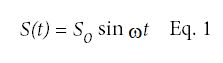Where S(t) is the stress at time, t; So is the maximum stress or stress amplitude; and ω is the frequency of oscillation. The strain response will depend on the viscous and elastic behavior of the sample and is governed by Eq. 2, where e(t) is the elastic strain at time, t, eo is the maximum strain or strain amplitude and θ is the phase shift between the stress-time and strain-time waveform.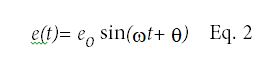For purely elastic or hookean materials, the phase shift, θ=0 and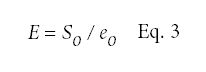Where E = Modulus of Elasticity. For purely viscous materials the phase shift, θ = ϖ /2 and the Modulus of Elasticity is undefined. Eq. 2 represents the elastic strain response to the sinusoidally varying stress. The phase angle, θ, between two sinewaves of equal period, T, is given by: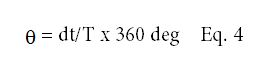Where, dt=time shift between the stress-time and strain-time waveforms and T = period of oscillation.

Trigonometry allows us to rewrite Eq. 2 as: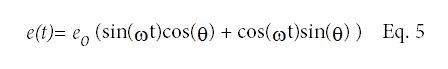And the in-phase elastic strain is given by: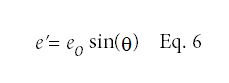And the out of phase imaginary viscous strain is given by: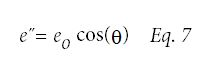The vector sum of the in-phase and out-of-phase strains results in a complex strain, e*.To summarize dynamic mechanical analysis, a sample is subjected to a sinusoidally varying stress of amplitude, So, and frequency, f, or period, T (1/f), and the amplitude, eo, and time shift, dt, of the resulting strain waveform is measured. From this, the phase lag or loss angle, θ, is calculated from Eq. 4 and the elastic or storage modulus, Eʹ, is given by: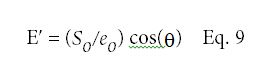The amount of energy lost due to friction and internal motions, called the loss modulus, E˝, is given by: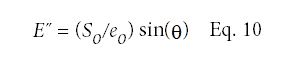The tangent of the phase lag or loss angle, tan(θ), is called the loss tangent or damping factor and provides a measure of how much energy is lost due to the viscous nature of the material. From Figure 5, we can determine the following viscoelastic properties of the polypropylene sample.

dt = 0.012 sec

T = 0.1 sec (10 Hz)

So = (12.521 – 4.779)/2 = 3.871 Mpa

eo = (1.0244-0.6049)/(2 x 100) = 0.00209 mm/mm

Using Eqs 4, 9 and 10, the loss angle, storage modulus and loss modulus are calculated as:

q = 0.012/0.1 x 360 = 43.2 deg

Eʹ = 3.871/0.00209 x cos (43.2) = 1,350 Mpa

E˝ = 3.871/0.00209 x sin (43.2) = 1,268 MPa

In addition, the loss tangent, tan(θ) = tan(43.2) = 0.939.

ASTM D638 Standard Test Method for Tensile Properties of Plastics governs the determination of Young’s Modulus for polypropylene. Figure 6 is the stress-strain diagram of the polypropylene sample pulled in tension to failure. From Figure 6, Young’s Modulus of Elasticity is calculated to be:

E = 1,884 MPa

In theory the storage modulus, E’ and Young’s Modulus, E, should be equal. However, Young’s Modulus is calculated by continuously pulling a sample to failure and using a range of stress and strain values in the elastic region, whereas the storage modulus is calculated with a pair of stress-strain values at a given point in the elastic region under oscillating loads.

A brief history and introduction to dynamic mechanical analysis and the viscoelasticity of materials has been given. DMA has been shown to be a simple and efficient means to determine the mechanical behavior of polymers and plastics over a range of temperatures and frequencies. Even though DMA has been employed for close to a century, it is not well understood by those performing mechanical tests. Furthermore, undergraduate engineering curriculum provide little exposure to DMA testing and the viscoelastic properties of materials. However, the development of new materials and diagnostic instruments will require a greater understanding of viscoelasticity and how to measure its behavior. Q

#### References:

Zhang, G., Evaluating the viscoelastic properties of biologic tissues in a new way. J Musculoskelet Neuronal Interact. 2005;5(1):85-90.

Palcio-Torralba, Javier, Hammer, Steven, Good, Daniel W., McNeill, Alan, S., Stewart, Grant D., Reuben, Robert L., Chen, Yuhang, Quantitative diagnostics of soft tissue through viscoelastic characterization using time-based instrumented palpation. Journal of the Mechanical Behavior of Biomedical Materials, 41, 2015:149-160.

Ferry J., Viscoelastic Properties of Polymers. John Wiley & Sons, New York: 1980.

Findley, William N., Lai, James S., Onaran, Kasif, Creep and Relaxation of Non-Linear Viscoelastic Materials. With an Introduction to Linear Viscoelasticity. North Holland Publishing Co. 1976.

Menard, Kevin P., Dynamic Mechanical Analysis, A Practical Introduction, 2nd Edition, Taylor and Francis Group LLC, 2008.

Hylton, Donald C., Understanding Plastics Testing, Hanser Gardner Publications, Inc. 2004.

Park, Joon, Lakes, R.S., Biomaterials – An Introduction, 3rd Edition, Springer, 2010.